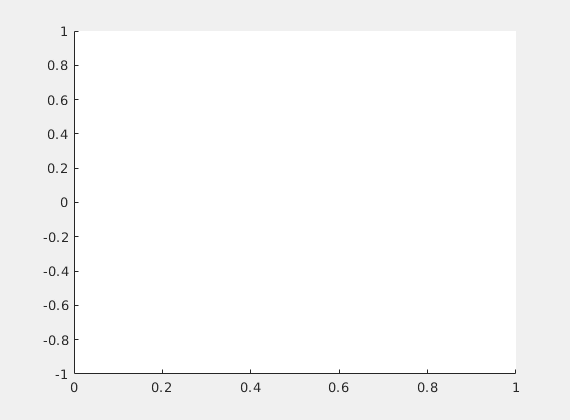# Control of Fractional Heat Equation

We use DyCon Toolbox for solving numerically the following control problem: given any $T>0$, find a control function $g\in L^2( ( -1 , 1) \times (0,T))$ such that the corresponding solution to the parabolic problem

satisfies $z(x,T)=0$.

Here, for all $s\in(0,1)$, $(-d_x^2)^s$ denotes the one-dimensional fractional Laplace operator, defined as the following singular integral

## Discretization of the problem

As a first thing, we need to discretize \eqref{frac_heat}. Hence, let us consider a uniform N-points mesh on the interval $(-1,1)$.

N = 50;
xi = -1; xf = 1;
xline = linspace(xi,xf,N+2);
xline = xline(2:end-1);


Out of that, we can construct the FE approxiamtion of the fractional Lapalcian, using the program FEFractionalLaplacian developped by our team, which implements the methodology described in .

s = 0.8;
A = -FEFractionalLaplacian(s,1,N);
M = massmatrix(xline);


Moreover, we build the matrix $B$ defining the action of the control, by using the program “BInterior” (see below).

a = -0.3; b = 0.8;
B = BInterior(xline,a,b,'Mass',true);


We can then define a final time and an initial datum

FinalTime = 0.5;
Y0 =sin(pi*xline);


and construct the system

dynamics = pde('A',A,'B',B,'InitialCondition',Y0,'FinalTime',FinalTime,'Nt',100);
dynamics.mesh = xline;
dynamics.MassMatrix = M;
solve(dynamics);

Error using ode/set.InitialCondition (line 353)
The Initial Condition must be a matrix: [50x1]

Error in ode (line 264)
obj.InitialCondition = p.Results.InitialCondition;

Error in pde (line 1)
classdef pde < ode

dynamics = pde('A',A,'B',B,'InitialCondition',Y0,'FinalTime',FinalTime,'Nt',100);


Y = dynamics.StateVector.Symbolic;
U = dynamics.Control.Symbolic;


## Construction of the control problem

Secondly, we construct the control problem, which consists in minimizing the functional

In this case, we choose the classical HUM functional in which

and

Moreover, we set the final target to $y(T)=0$.

dx = xline(2)-xline(1);
YT = 0.0*xline;
epsilon = dx^4;
Psi  = @(T,Y)   (1/(2*epsilon))*dx*(YT.' - Y).'*(YT.' - Y);
L    = @(t,Y,U) (1/2)*dx*(U.'*U);
iCP1 = Pontryagin(dynamics,Psi,L);


## Solution of the minimization problem

As a final step, we use the gradient method we developed for solving the minimization problem and computing the control. In this case, we choose to use the Adaptive Gradient Descent algorithm.

tol = 1e-4;

U0 = dynamics.Control.Numeric;
%% [Uopt , JOpt] = fminunc(@(U) Control2Functional(iCP1,U),U0,options)

f0 = dynamics.InitialCondition*0;


As we see, the algorithm has stopped since it has reached the maximum number of iterations allowed, and not because it has encountered a minimum of the functional $J$.

Actually, we can see in the figure below that the final state is not controlled to zero.

%%plot(iCP1)


This is because the HUM functional $J$ we chose to minimize is not suitable for numerical implementation. Indeed, as it has been pointed out in , even though $J$ has a unique minimizer, it can be a difficult task to compute it numerically.

Hence, it is convenient to deal with a penalized version of our optimization problem, applying the well-known Penalized Hilbert uniqueness method. This will be the scope of a future post.

solve(dynamics)
dynamics.label = 'Free';
iCP1.Dynamics.label = 'Control';


animation([iCP1.Dynamics,dynamics],’YLim’,[-1 1],’xx’,0.05)function M = massmatrix(mesh)
N = length(mesh);
dx = mesh(2)-mesh(1);
M = 2/3*eye(N);
for i=2:N-1
M(i,i+1)=1/6;
M(i,i-1)=1/6;
end
M(1,2)=1/6;
M(N,N-1)=1/6;

M=dx*sparse(M);

end


 U. Biccari and V. Hern'andez-Santamar'ia - \textit{Controllability of a one-dimensional fractional heat equation: theoretical and numerical aspects}, IMA J. Math. Control. Inf., to appear